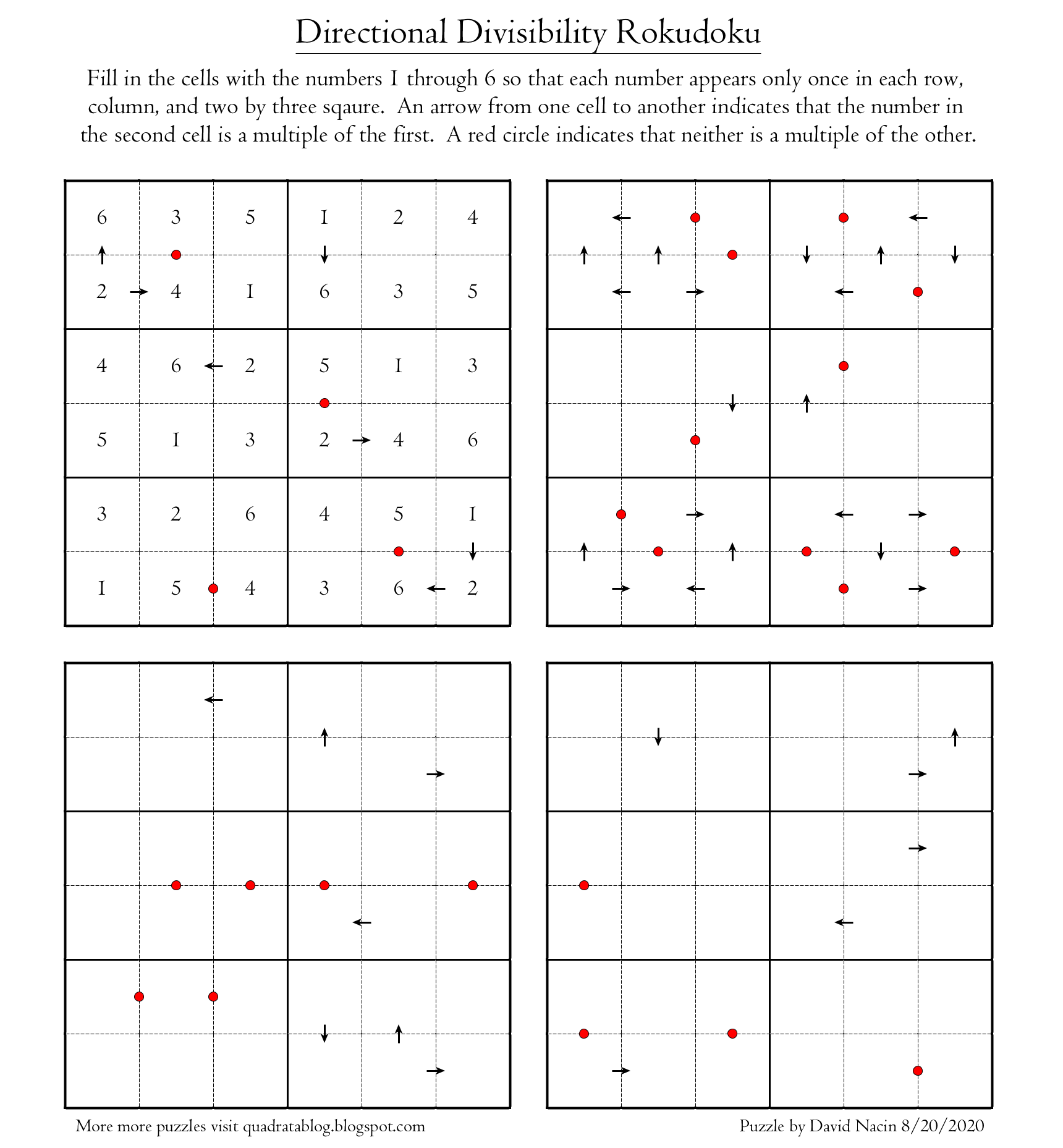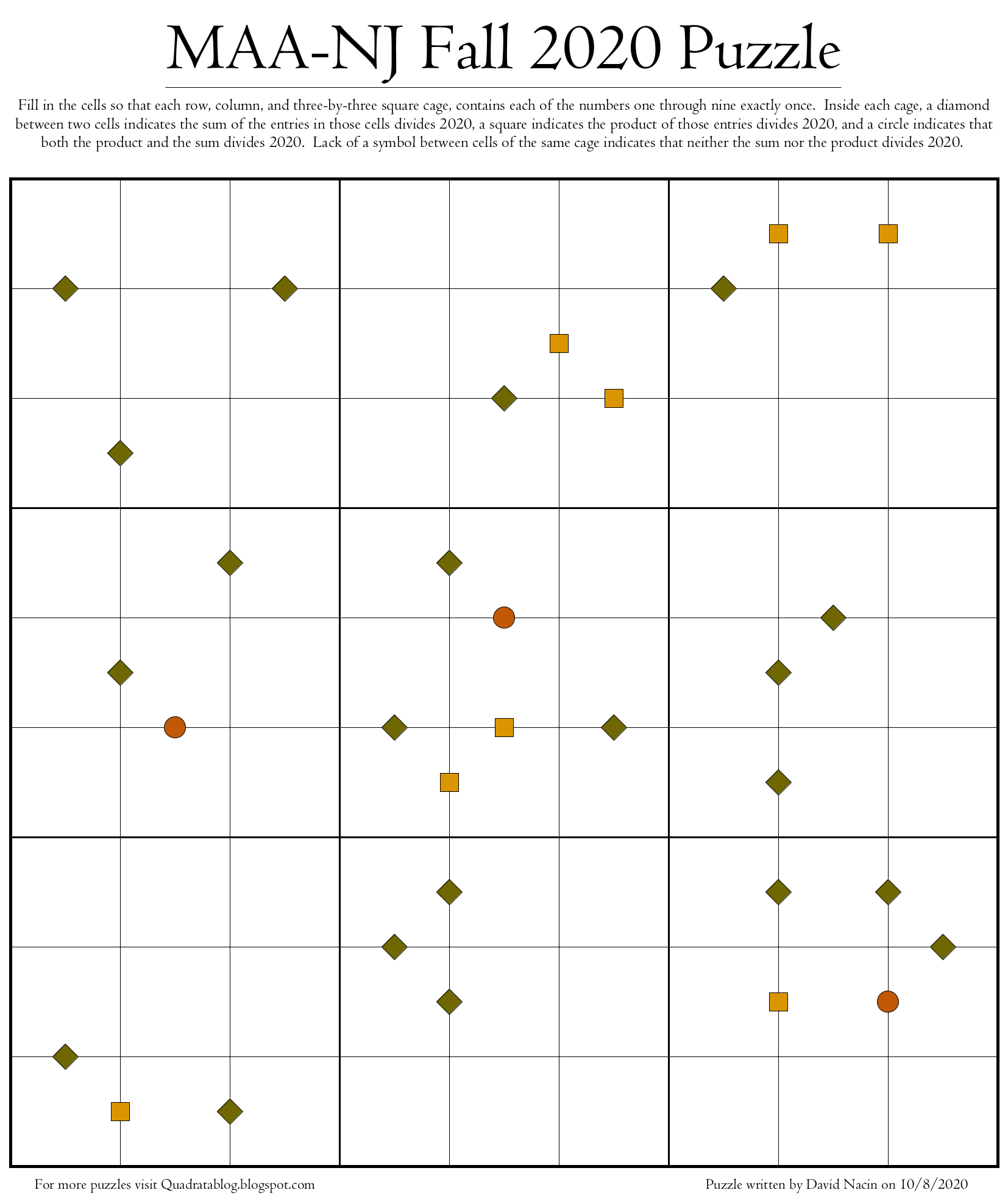## Quadrata: A Free Puzzle Blog

Links to puzzle sites all around the web
Posts: 8
Joined: Wed 12 Aug, 2020 6:20 pm

### Quadrata: A Free Puzzle Blog

Just wanted to let everyone here know about a puzzle website that I maintain. I post new original puzzle varieties there about twice a month. Today I posted three Sudoku variants where the clues are the greatest common divisors of the numbers in adjacent cells. This puzzle is below, and the solution and more info can be found at the blog at:Posts: 8
Joined: Wed 12 Aug, 2020 6:20 pm

### Re: Quadrata: A Free Puzzle Blog

Here are some small puzzles based around divisibility, with arrows revealing which number divides which. Dots indicate that neither divides the other. The first puzzle is solved to give you a visual instruction. The next three puzzles increase with difficulty as they go.Posts: 8
Joined: Wed 12 Aug, 2020 6:20 pm

### Re: Quadrata: A Free Puzzle Blog

Here's a 9x9 Sudoku where the clues tell you what the sum is of the two adjacent cells. The catch is that the numbers are only given when they are even. Thus a numberless dot reveals that the sum is odd without telling you what that sum is.Solution, a full PDF and more can be found at: https://quadratablog.blogspot.com/2020/ ... udoku.html

Posts: 8
Joined: Wed 12 Aug, 2020 6:20 pm

### Re: Quadrata: A Free Puzzle Blog

Here are some smaller 6x6 versions of my last puzzle, the even sum Sudoku. They start easy and get harder as they go. Rules are on the puzzle and the solution and PDF can be found at my blog here:Posts: 8
Joined: Wed 12 Aug, 2020 6:20 pm

### Re: Quadrata: A Free Puzzle Blog

Here's a Sudoku with clues based around the products of numbers in adjacent cells. Even products are fully revealed but odd products result in a numberless dot.

Solution and more at: https://quadratablog.blogspot.com/2020/ ... udoku.htmlPosts: 8
Joined: Wed 12 Aug, 2020 6:20 pm

### Re: Quadrata: A Free Puzzle Blog

Here's a puzzle I designed for a math conference that took place last weekend. You don't need to know much more than which numbers divide 2020 to attempt it, so I figured I would share it here as well. For more details and a solution visit my blog here: https://quadratablog.blogspot.com/2020/ ... uzzle.htmlPosts: 8
Joined: Wed 12 Aug, 2020 6:20 pm

### Re: Quadrata: A Free Puzzle Blog

This next puzzle is a little mathy in the sense that it uses the primes, but really all you need to know is that the first three prime numbers are 2, 3, and 5. Basically, if you see a number between cells then exactly one of those cells contains a prime, and that number tells you the sum. A diamond means that either neither of the numbers or both of the numbers must be prime. Other than that, the rules are identical to normal Sudoku with each number appearing exactly once in every row, column, and two-by-three cage.

For a solution, PDF, and zoomed in individual puzzles visit Quadrata at www.quadratablog.blogspot.com.